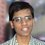# A problem on Floor and Ceiling Functions!

Is it true or false?

$\large{\left \lceil \dfrac{1 + \sqrt{1+8n}}{2} \right \rceil - 1 = \left \lfloor \sqrt{2n} \right \rfloor}$

Here $n$ is a positive integer.

If it is false, provide me a counter-example. If it's true, please provide me a proof.Note by Satyajit Mohanty
6 years ago

This discussion board is a place to discuss our Daily Challenges and the math and science related to those challenges. Explanations are more than just a solution — they should explain the steps and thinking strategies that you used to obtain the solution. Comments should further the discussion of math and science.

When posting on Brilliant:

• Use the emojis to react to an explanation, whether you're congratulating a job well done , or just really confused .
• Ask specific questions about the challenge or the steps in somebody's explanation. Well-posed questions can add a lot to the discussion, but posting "I don't understand!" doesn't help anyone.
• Try to contribute something new to the discussion, whether it is an extension, generalization or other idea related to the challenge.

MarkdownAppears as
*italics* or _italics_ italics
**bold** or __bold__ bold
- bulleted- list
• bulleted
• list
1. numbered2. list
1. numbered
2. list
Note: you must add a full line of space before and after lists for them to show up correctly
paragraph 1paragraph 2

paragraph 1

paragraph 2

[example link](https://brilliant.org)example link
> This is a quote
This is a quote
    # I indented these lines
# 4 spaces, and now they show
# up as a code block.

print "hello world"
# I indented these lines
# 4 spaces, and now they show
# up as a code block.

print "hello world"
MathAppears as
Remember to wrap math in $$ ... $$ or $ ... $ to ensure proper formatting.
2 \times 3 $2 \times 3$
2^{34} $2^{34}$
a_{i-1} $a_{i-1}$
\frac{2}{3} $\frac{2}{3}$
\sqrt{2} $\sqrt{2}$
\sum_{i=1}^3 $\sum_{i=1}^3$
\sin \theta $\sin \theta$
\boxed{123} $\boxed{123}$

Sort by:

False. Often, they don't agree.

- 6 years ago

I'm sorry. I forgot to mention that $n$ is a positive integer! Can you find some counter-example now?

- 6 years ago

..

$\dfrac { 1 }{ 2 } (1+\sqrt { 1+8\cdot 4 } )-1=2.37228...$
$\sqrt { 2\cdot 4 } =2.828427...$

But because of the way ceiling and floor functions work, these two go off in opposite directions. This is just one example.

- 6 years ago

if n = 2 it is not true

the only solutions are: 0,1,3,4,6

- 6 years ago

It is true for $n=2$. I don't agree!

- 6 years ago

[] means floor?

- 6 years ago

Look. $\lceil . \rceil$ means ceiling and $\lfloor . \rfloor$ means floor.

- 6 years ago

Ah- i see =)

- 6 years ago

It's not true when n = 12 (calculated with Wolfram Alpha)

- 6 years ago

It's not true when n = 12 (calculated with Wolfram Alpha)

- 6 years ago

Hint: Consider what happens when $k^2 \leq 2n < (k+1)^2$.

Staff - 6 years ago

Thanks :) I got it. I actually had a doubt on a solution to this problem: Peculiar Sequence of Positive Integers! as the generalized version of my solution did not match the generalized version of the other solution.

- 6 years ago

If you see my note, I was pointing out the mistake that @Chew-Seong Cheong made in the generalization.

The true version of the statement that you are looking for, is

${\left \lceil \dfrac{1 + \sqrt{1+8n}}{2} \right \rceil = \left \lfloor \sqrt{2n} + \frac{3}{2} \right \rfloor}$

Staff - 6 years ago